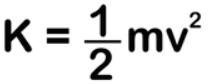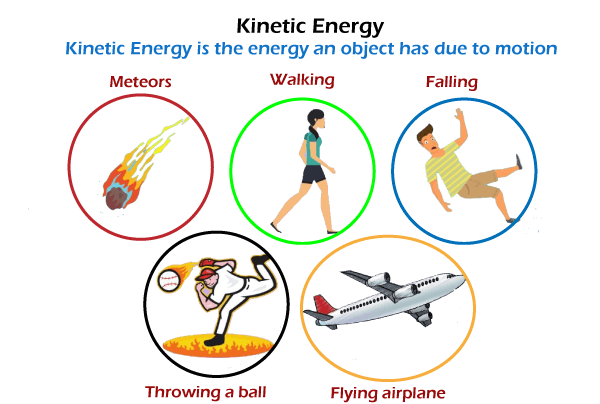Swati Talwar

HS-PS1-3

# Kinetic Energy In Chemistry Study Guide

Energy exists in both fixed and dynamic states of matter.

## INTRODUCTION

It is nearly impossible to catch a squirrel while it is in action. On top of their flexible small bodies, their motions are exceedingly rapid. They are often characterized to be 'full of energy.

Unlike our many other everyday statements, this is surprisingly correct on chemical and physical levels. The definition of kinetic energy in chemistry, of an object, is the measure of the work it does due to its motion. Kinetic energy is used in everyday activities such as walking, climbing, tossing, and dropping.Source

### KINETIC ENERGY

The capacity to do work or produce heat is defined as energy.

We must apply force to accelerate an object, and we must work in order to apply force. Energy is transferred when work is done on an object, and the object moves at a new constant speed. The energy transferred is known as kinetic energy, and it is determined by the mass and speed reached.

### HOW IS KINETIC ENERGY MEASURED?

Kinetic energy is a scalar quantity that can only be defined in terms of magnitude. The kinetic energy is measured in Joule (J), which is equivalent to 1 kg.m².s-².

The kinetic energy equation is given as:Where K is the kinetic energy, m is the body's mass, and v is the body's velocity.

### EXAMPLES OF KINETIC ENERGY

Because of its mass, a truck travelling down the road has much more kinetic energy than a car travelling at the same velocity.

Because water has a specific velocity and mass, a river moving at a certain speed contains kinetic energy.Source

### POTENTIAL ENERGY

Potential energy is defined as the energy that an object possesses due to its position relative to other objects, internal tensions, electric charge, or other causes.

When a spring is pushed from its equilibrium position, it gains energy, which we notice as stress on our hands when we stretch it. Potential energy is defined as a type of energy that results from a change in its location or state. The SI unit of potential energy is kg m² / s² and is measured in Joule (J).

The general formula of potential energy is (Potential Energy) W = m.g.h, where m is the mass, g is the acceleration due to gravity, and h is the height.

## CONCLUSION:

• The capacity to do work or produce heat is defined as energy.
• Kinetic energy is the energy released as a result of motion.
• Potential energy is the amount of energy that is stored in materials.
• The SI unit of energy is kg m² / s² and is measured using Joule (J).

### FAQs:

1. How do you calculate kinetic energy in chemistry?

Kinetic energy can be calculated using the formulae KE = 1/2 mv²

2. What are three kinetic energy examples?

The three examples of kinetic energy are:

• A river flowing at a certain speed
• The energy of an asteroid falling towards earth
• A speeding bullet

We hope you enjoyed studying this lesson and learned something cool about Chemical Kinetic Energy! Join our Discord community to get any questions you may have answered and to engage with other students just like you! Don't forget to download our App to experience our fun VR classrooms - we promise it makes studying much more fun! 😎

## SOURCES:

1. Types of Energy: https://courses.lumenlearning.com/introchem/chapter/types-of-energy/. Accessed 22 Feb 2022.
2. Kinetic Energy: https://www.ck12.org/c/chemistry/kinetic-energy/lesson/SI-Kinetic-Energy-Units-CHEM/. Accessed 22 Feb 2022.